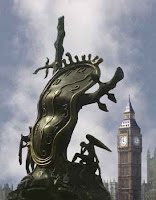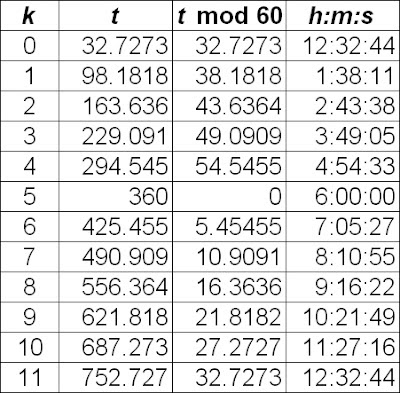## Sunday, May 06, 2007

### Straight talk on Marilyn's clockA question of time

The aptly named Marilyn vos Savant is a very clever lady, but not a mathematician. She did show up several indignant mathematicians who disagreed with her analysis of the Monty Hall problem—she was indeed correct—but then she dramatically exceeded her grasp when she pontificated about Fermat's Last Theorem. She later retracted her unfounded criticisms of the Andrew Wiles proof. I think that was a lesson learned.

Marilyn continues to like clever math puzzles and she's good at them. In today's Parade, Marilyn presents a riddle concerning time. Here is the original question and her answer to it:
What time is missing, and what do these signify: 1:38, 2:44, 3:49, 4:55, ——, 7:05, 8:11, 9:16, 10:22, 11:27, 12:33?

Jacob Miller, Mount Joy, Pa.

Answer: At these times, the hour and minute hands form a straight line, so 6:00 is missing.
That seemed simple enough, didn't it?

Of course, 6:00 is the only time in Marilyn's list when the alignment of the hour and minute hands is readily apparent. How were the other times puzzled out?

Let's do a little math.

Parametric equations solve the problem nicely. Let h(t) be the location of the hour hand and m(t) be the location of the minute hand. Assume that both are at 0 (or twelve!) at time t = 0, where we'll count off t in minutes. Since the minute hand travels through all sixty minutes of the clock face in the time that it takes the hour hand to move through five minutes, it travels 12 times as fast. Thus we have h(t) = t/12 and m(t) = t.

Since we're starting with the clock hands positioned at 12:00, the first clock-time that we'll encounter from Marilyn's list is 12:33. Is that correct? How accurate is the answer?

The key fact is that the positions of the hands will differ by 30, so we can substitute and solve for t:

m(t) − h(t) = 30
tt/12 = 30
11t/12 = 30
t = 360/11 ≈ 32.72

If we convert t into minutes and seconds, we have something very close to 32 minutes and 44 seconds. In other words, the hands of the clock do not form a straight line at precisely 12:33. They form a straight line at 12:32:44. I think we can agree that, for most purposes, 12:33 is a perfectly acceptable answer.

How about all the other times? Given the rate at which the minute hand outstrips the hour hand, the difference of 30 that we used in the above calculation is valid only for our first computation. The more general criterion is that we want the positions of the hands to differ by odd multiples of 30. In other words, this is really a problem in modular arithmetic. (Isn't it an amusing coincidence that modular arithmetic is often introduced to students as “clock” arithmetic? No, it's not a coincidence at all!)

We already know that the difference between the positions of the minute and hour hands is given by 11t/12, so the general equation we need to solve is

11t/12 = (2k + 1)30,

where k is an integer. We have already solved the case where k = 0.

The general solution is

t = (2k + 1)360/11.

We just need to crank out a t value for each choice of k, remembering that k = 0 corresponds to the noon hour. We also need to reduce our t values modulo 60, so that they correspond to actual clock times. Then we convert the fractional part of t into seconds, rounding to the nearest second. Here are the results, which you may wish to check for yourself:There are a couple of interesting features to our results. First, there is no solution that corresponds to the 5:00 hour (unless you want to argue in favor of 5:60). That's when we hit the special case of 6:00. Also, if we try to find twelve solutions by computing the result for k = 11, we find ourselves back where we started from, looking at 12:32:44 again.

I see by the clock that we're out of time, so that's all for today, kids!